Download Presentation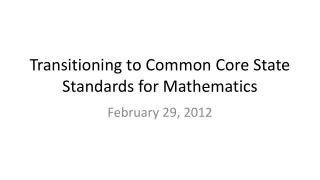Transitioning to Common Core State Standards for Mathematics

# Transitioning to Common Core State Standards for Mathematics - PowerPoint PPT Presentation

Transitioning to Common Core State Standards for Mathematics. February 29, 2012. Mathematics PD Plan. Face-to-Face Online Blended. Mathematics PD Plan. Cognitively Guided Instruction Extending Children ’ s Mathematics. Mathematics PD Plan.I am the owner, or an agent authorized to act on behalf of the owner, of the copyrighted work described.
Download Presentation## Transitioning to Common Core State Standards for Mathematics

An Image/Link below is provided (as is) to download presentation

Download Policy: Content on the Website is provided to you AS IS for your information and personal use and may not be sold / licensed / shared on other websites without getting consent from its author.While downloading, if for some reason you are not able to download a presentation, the publisher may have deleted the file from their server.

- - - - - - - - - - - - - - - - - - - - - - - - - - E N D - - - - - - - - - - - - - - - - - - - - - - - - - -
Presentation Transcript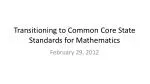### Transitioning to Common Core State Standards for Mathematics

February 29, 2012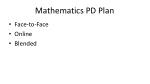Mathematics PD Plan
• Face-to-Face
• Online
• Blended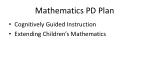Mathematics PD Plan
• Cognitively Guided Instruction
• Extending Children’s Mathematics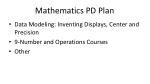Mathematics PD Plan
• Data Modeling: Inventing Displays, Center and Precision
• 9-Number and Operations Courses
• Other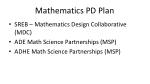Mathematics PD Plan
• SREB – Mathematics Design Collaborative (MDC)
• ADE Math Science Partnerships (MSP)
• ADHE Math Science Partnerships (MSP)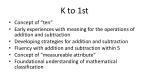K to 1st
• Concept of “ten”
• Early experiences with meaning for the operations of addition and subtraction
• Developing strategies for addition and subtraction
• Fluency with addition and subtraction within 5
• Concept of “measureable attribute”
• Foundational understanding of mathematical classification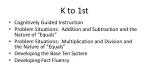K to 1st
• Cognitively Guided Instruction
• Problem Situations: Addition and Subtraction and the Nature of “Equals”
• Problem Situations: Multiplication and Division and the Nature of “Equals”
• Developing the Base Ten System
• Developing Fact Fluency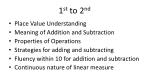1st to 2nd
• Place Value Understanding
• Meaning of Addition and Subtraction
• Properties of Operations
• Strategies for adding and subtracting
• Fluency within 10 for addition and subtraction
• Continuous nature of linear measure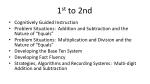1st to 2nd
• Cognitively Guided Instruction
• Problem Situations: Addition and Subtraction and the Nature of “Equals”
• Problem Situations: Multiplication and Division and the Nature of “Equals”
• Developing the Base Ten System
• Developing Fact Fluency
• Strategies, Algorithms and Recording Systems: Multi-digit Addition and Subtraction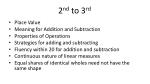2nd to 3rd
• Place Value
• Meaning for Addition and Subtraction
• Properties of Operations
• Strategies for adding and subtracting
• Fluency within 20 for addition and subtraction
• Continuous nature of linear measures
• Equal shares of identical wholes need not have the same shape2nd to 3rd
• Cognitively Guided Instruction
• Problem Situations: Addition and Subtraction and the Nature of “Equals”
• Problem Situations: Multiplication and Division and the Nature of “Equals”
• Developing the Base Ten System
• Developing Fact Fluency
• Strategies, Algorithms and Recording Systems: Multi-digit Addition and Subtraction
• Fraction Concepts: Equal Sharing3rd to 4th
• Meaning for Operations
• Properties of Operations
• Strategies for Operations
• Fluency for adding and subtracting within 20
• Fluency for multiplying and dividing within 100
• Fraction meaning
• Continuous nature of linear measure3rd to 4th
• Cognitively Guided Instruction/Extending Children’s Mathematics
• Problem Situations: Addition and Subtraction and the Nature of “Equals”
• Problem Situations: Multiplication and Division and the Nature of “Equals”
• Developing the Base Ten System
• Developing Fact Fluency
• Strategies, Algorithms and Recording Systems: Multi-digit Addition and Subtraction
• Fraction Concepts: Equal Sharing
• Other Problem Situations: Multiplication and Division4th to 5th
• Meaning of Operations
• Properties of Operations
• Strategies for performing operations
• Fluency with basic facts
• Place Value
• Fraction meaning and equivalence
• Angles4th to 5th
• Extending Children’s Mathematics
• Problem Situations: Multiplication and Division and the Nature of “Equals”
• Other Problem Situations: Multiplication and Division
• Developing the Base Ten System
• Strategies, Algorithms and Recording Systems: Multi-digit Addition and Subtraction
• Strategies, Algorithms and Recording Systems: Multi-Digit Multiplication and Division
• Fraction Concepts: Equal Sharing
• Fraction Operations: Multiple Grouping5th to 6th
• Fraction meaning
• Fraction Operations
• Geometric Classification
• Extension of place value to decimal notation
• Lingering concerns…Understanding of operations, strategies for operations and fluency5th to 6th
• Extending Children’s Mathematics
• Fraction Concepts: Equal Sharing
• Fraction Operations: Multiple Grouping
• Problem Situations: Multiplication and Division and the Nature of “Equals”
• Other Problem Situations: Multiplication and Division
• Developing the Base Ten System
• Strategies, Algorithms and Recording Systems: Multi-digit Addition and Subtraction
• Strategies, Algorithms and Recording Systems: Multi-Digit Multiplication and Division6th to 7th
• Single Variable Data
• Proportional reasoning
• Geometric measures: perimeter, area, surface area and volume
• Independent and dependent variables
• Extending knowledge of number to negative values
• Lingering concerns: understanding rational numbers meaning of operations, strategies for operations, fluency6th to 7th
• Extending Children’s Mathematics
• Fraction Concepts: Equal Sharing
• Fraction Operations: Multiple Grouping
• Problem Situations: Multiplication and Division and the Nature of “Equals”
• Other Problem Situations: Multiplication and Division
• Developing the Base Ten System
• Strategies, Algorithms and Recording Systems: Multi-Digit Multiplication and Division
• Data Modeling: Inventing Displays, Center and Precision7th to 8th
• Proportional Reasoning and its application
• Rational number concepts and computation
• Statistics and Probability
• Writing and solving equations7th to 8th
• Data Modeling: Inventing Displays, Center and Precision
• Other Coop offeringsGrade 8 and Beyond

8th Grade Mathematics becomes more like what Algebra I is under the Arkansas Mathematics Frameworks.

• Extends rational number system to the real numbers
• Function Concepts
• Intense study of linear functions
• Congruence, Similarity and Pythagorean TheoremGrade 8 and Beyond

Algebra I will finalize linear functions, but most of the instruction will focus on quadratic and/or exponential functions.

Algebra II will look a great deal more like what pre-calculus has been under the Arkansas Frameworks.